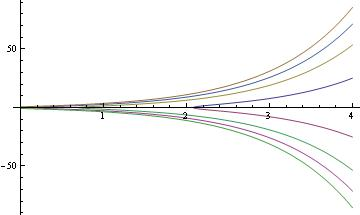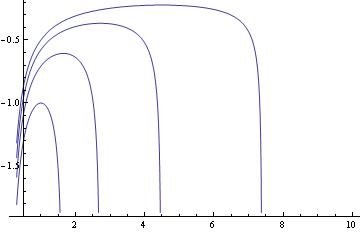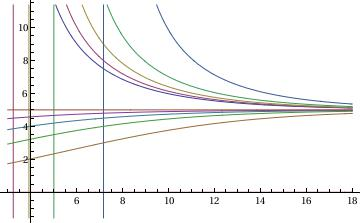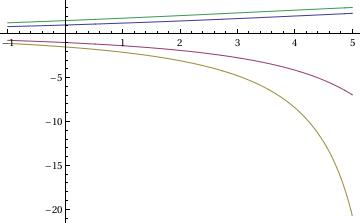# Preface

A Bernoulli differential equation is an equation of the form $$y' + a(x)\,y = g(x)\,y^{\nu} ,$$ where a(x) are g(x) are given functions, and the constant ν is assumed to be any real number other than 0 or 1. Bernoulli equations have no singular solutions.

# Bernoulli Equations

A differential equation

$y' + p(x)\,y = g(x)\,y^\alpha ,$
where α is a real number not equal to 0 or 1, is called a Bernoulli differential equation. It is named after Jacob (also known as James or Jacques) Bernoulli (1654--1705) who discussed it in 1695. Jacob Bernoulli was born in Basel, Switzerland. Following his father's wish, he studied theology and entered the ministry. But contrary to the desires of his parents, he also studied mathematics and astronomy. He traveled throughout Europe from 1676 to 1682, learning about the latest discoveries in mathematics and the sciences under leading figures of the time. His travels allowed him to establish correspondence with many leading mathematicians and scientists of his era, which he maintained throughout his life.

Bernoulli returned to Switzerland and began teaching mechanics at the University in Basel from 1683. In 1684 he married Judith Stupanus; and they had two children. He was appointed professor of mathematics at the University of Basel in 1687, remaining in this position for the rest of his life. By that time, he had begun tutoring his brother Johann Bernoulli on mathematical topics. The two brothers began to study the calculus as presented by Leibniz in his 1684 paper on the differential calculus. Jacob collaborated with his brother on various applications of calculus. However the atmosphere of collaboration between the two brothers turned into rivalry as Johann's own mathematical genius began to mature, with both of them attacking each other in print, and posing difficult mathematical challenges to test each other's skills. By 1697, the relationship had completely broken down.

Jacob Bernoulli is known for his numerous contributions to calculus, and along with his brother Johann, was one of the founders of the calculus of variations. In May 1690, in a paper published in Acta Eruditorum, Jacob Bernoulli showed that the problem of determining the isochrone is equivalent to solving a first-order nonlinear differential equation. The isochrone, or curve of constant descent, is the curve along which a particle will descend under gravity from any point to the bottom in exactly the same time, no matter what the starting point. He also discovered the fundamental mathematical constant e, which Euler later denoted by e.

Jacob made a great contribution to calculus. By 1689 he had published important work on infinite series and showed that harmonic series diverges, which had actually been proved by Mengoli 40 years earlier. Jacob Bernoulli also discovered a general method to determine evolutes of a curve as the envelope of its circles of curvature. He also investigated caustic curves and in particular he studied these associated curves of the parabola, the logarithmic spiral and epicycloids around 1692. The lemniscate of Bernoulli was first conceived by Jacob Bernoulli in 1694. In 1695 he investigated the drawbridge problem which seeks the curve required so that a weight sliding along the cable always keeps the drawbridge balanced.

However, his most important contribution was in the field of probability, where he derived the first version of the law of large numbers in his work Ars Conjectandi, published in Basel in 1713, eight years after his death.

Jacob Bernoulli chose a figure of a logarithmic spiral (its equation in polar coordinates is $$r = a\, e^{b\,\theta}$$ ) and the motto Eadem mutata resurgo ("Changed and yet the same, I rise again") for his gravestone; the spiral executed by the stonemasons was, however, an Archimedean spiral, $$r = a + b\, \theta .$$

Bernoulli equations are special because they are nonlinear differential equations with known exact solutions. Moreover, they do not have singular solutions---similar to linear equations. There are two methods known to determine its solutions: one was discovered by himself, and another is credited to Gottfried Leibniz (1646--1716).

Leibniz substitution

The Bernoulli equation $$y' + p(x)\,y = g(x)\,y^\alpha$$ can be reduced to a linear differential equation with substitution

$u= y^{1 - \alpha} .$
Then for u we obtain a linear equation
$u' + \left(1 - \alpha \right) p(x)\, u = \left(1 - \alpha \right) g(x) .$

This method is implemented in Mathematica:
y=x^(1-n)
L[x_] := D[x, t] + p*x - q*x^n;
x = y[t]^(1/(1 - n));
a2 = Simplify[L[x]];
a3 = a2/y[t]^(n/(1 - n));
Simplify[PowerExpand[a3]]
Out= ConditionalExpression[
8^(n/(1 - n)) t^((2 n)/(3 - 3 n)) (7 + t^(8/3))^(
n/(-1 + n)) (p (7/(8 t^(2/3)) + t^2/8)^(1/(1 - n)) -
q (7/(8 t^(2/3)) + t^2/8)^(n/(1 - n)) - (
2 (7/(8 t^(2/3)) + t^2/8)^(1/(1 - n)) (-7 + 3 t^(8/3)))/(
3 (-1 + n) t (7 + t^(8/3)))), (Re[t] >= 0 ||
t $NotElement] Reals) && (Re[u] >= 0 || u \[NotElement] Reals)] Bernoulli Method The Bernoulli equation $$y' + p(x)\,y = g(x)\,y^\alpha$$ can be reduced to two separable equations. We seek its solution as the product of two functions $$y(x) = u(x)\,v(x) ,$$ where u(x) is a solution (we need just one of them) of a linear part: \[ u' + p(x)\,u = 0 \qquad \Longrightarrow \qquad \frac{{\text d}u}{u} = - p(x)\,{\text d}x .$
So upon its integration, we have
$u(x) = \exp \left\{ - \int p(x)\,{\text d}x \right\} .$
The second multiple, v(x), is the general solution of the "truncated" Bernoulli equation without a linear part:
$v' = g(x)\,u^{\alpha -1} \,v^\alpha \qquad \Longrightarrow \qquad \frac{{\text d}v}{v^{\alpha}} = g(x)\,u^{\alpha -1} \,{\text d}x .$
This separable equation is also integrated without a problem:
$v^{1-\alpha} = \left( 1- \alpha \right) \int g(x)\,u^{\alpha -1} \,{\text d}x .$
Taking 1 - α root and multiplying the result by u(x) yields the required solution.

Example: Consider the differential equation $$y\, y' = y^2 + e^x .$$ To solve it, we first use the Leibniz substitution: $$u = y^{2} \quad \Longleftrightarrow \quad y = u^{1/2} .$$ Then $$y' = \frac{1}{2}\, u^{-1/2} u' \quad \Longrightarrow \quad y\,y' = \frac{1}{2}\, u'$$ and we get the linear differential equation

$\frac{1}{2}\, u' = u + e^x .$
To solve it, we first find an integrating factor by solving the corresponding separable equation
$\frac{1}{2}\, \mu' = -\mu \qquad \Longrightarrow \qquad \frac{{\text d} \mu}{\mu} = -2\,{\text d}x \qquad \Longrightarrow \qquad \mu (x) = e^{-2x} .$
Multiplying both sides of the equation $$u' - 2\,u = 2\, e^x$$ by the integrating factor, we obtain an exact equation
$\frac{\text d}{{\text d}x}\left( e^{-2x} u(x) \right) = 2\, e^{-x} \qquad \Longrightarrow \qquad e^{-2x} u(x) = C -2\, e^{-x} \qquad \Longrightarrow \qquad u (x) = C\,e^{2x} - 2\, e^x ,$
where C is a constant of integration. Returning to the original function, we find the general solution to the given differential equation $$y = \pm \sqrt{C\,e^{2x} - 2\, e^x} .$$

Now we demonstrate the application of the Bernoulli method by seeking the solution as the product y = u v, where u is a solution of the "linear part:"

$u' = u \qquad \Longrightarrow \qquad u (x) = e^{x} .$
Then v must be the general solution to the separable equation
$\left( u\, v \right) u\, v' = e^x \qquad \Longrightarrow \qquad v\, v' = e^{-x} .$
Separating variables and integrating, we obtain
$v\, {\text d}v = e^{-x} \, {\text d}x \qquad \Longrightarrow \qquad \frac{v^2}{2} = C- e^{-x} .$
Since $$v = \pm\sqrt{C- 2\,e^{-x}} ,$$ we multiply it by u to obtain the general solution. Now we use Mathematica:

curves = Flatten[
Table[{Sqrt[-2 E^x + c E^(2 x)], -Sqrt[-2 E^x + c E^(2 x)]}, {c,
1/4, 3, 3/4}]] (* increment is 3/4 *)
Out= {Sqrt[-2 E^x + E^(2 x)/4], -Sqrt[-2 E^x + E^(2 x)/4], Sqrt[-2 E^x +
E^(2 x)], -Sqrt[-2 E^x + E^(2 x)], Sqrt[-2 E^x + (7 E^(2 x))/
4], -Sqrt[-2 E^x + (7 E^(2 x))/4], Sqrt[-2 E^x + (5 E^(2 x))/
2], -Sqrt[-2 E^x + (5 E^(2 x))/2]}
Plot[Evaluate[curves], {x, 0, 4}, PlotRange -> All]Example: The four streamlines (corresponding to the values of an arbitrary constant C=1,2,3,4) from the general solution y=1/(x Sqrt[C-2 ln[x]]) of the Bernoulli equation
x y' =x^2 y^3 -y can be plotted with one command:

Plot[-1/(x Sqrt[#1 - 2 Log[x]] &) /@ {1, 2, 3, 4}, {x, .3, 10} ]Example: Consider the Bernoulli equation $$y' +x\,y=x\, y^4 .$$ Using Leibniz substitution

$u = y^{-3} \qquad \Longleftrightarrow \qquad y = u^{-1/3} \qquad \Longrightarrow \qquad y' = \frac{-1}{3}\,u^{-4/3} u' ,$
we reduce the given differential equation to a linear one.

$- \frac{1}{3}\, u^{-4/3} u' + x\, u^{-1/3} = x\, u^{-4/3} \qquad \Longrightarrow \qquad -u' + 3x\, u = 3x \qquad \Longrightarrow \qquad u' - 3x \, u = -3x .$
To find an integrating factor for the latter, we need to solve the separable equation
$\mu' + 3x\, \mu = 0 \qquad \Longrightarrow \qquad \frac{{\text d}\mu}{\mu} = -3x\, {\text d}x \qquad \Longrightarrow \qquad \mu (x) = e^{-3 x^2 /2} .$
Multiplication of the differential equation $$u' - 3x \, u = -3x$$ by μ yields an exact equation
$\frac{{\text d}}{{\text d}x} \left( \mu \, u \right) = -3x\, \mu \qquad \Longrightarrow \qquad \mu (x)\, u(x) = e^{-3 x^2 /2} +C \qquad \Longrightarrow \qquad u = 1 + C\, e^{3 x^2 /2} .$
Since $$y = u^{-1/3} ,$$ we obtain the general solution.
BerEq[x_,y_] := D[y[x],x]+x y[x] == y[x]^4
BNum =4
u[x_] == y[x]^(1-BNum)
yp = Solve[u'[x] == D[y[x]^(1-BNum),x],y'[x]]
LeftSide = Map[Function[t, t/y[x]^BNum],
BerEq[x, y] /. yp[] /. {(a_ == b_) -> a - b}]
L[x_, u_] = LeftSide /. {y[x]^(1 - BNum) -> u[x]}
SolU = DSolve[L[x,u] == 0,u[x],x]
Sol[x_] = Simplify[(u[x]/.SolU[]^(1/(1-BNum)))]
Out= 4
Out= u[x_] == 1/y[x]^3
Out= {{Derivative[y][x] -> -(1/3) y[x]^4 Derivative[u][x]}}
Out= -1 + x/y[x]^3 - Derivative[u][x]/3
Out= -1 + x u[x] - Derivative[u][x]/3
Out= {{u[x] ->
E^((3 x^2)/2) C -
E^((3 x^2)/2) Sqrt[(3 $Pi])/2] Erf[Sqrt[3/2] x]}} Out= u[x] /. {1/(u[x] -> 1/2 E^((3 x^2)/2) (2 C - Sqrt[6 \[Pi]] Erf[Sqrt[3/2] x]))^(1/3) } Now we find the general solution using the Bernoulli method: y = u*v, where u is a solution of the "truncated" linear equation $$u' + x\,u =0$$ and v is the general solution of the separable equation $$u\, v' = x\, \left( u\, v \right)^4 .$$ Solving the equation for u, we get \[ \frac{{\text d}u}{u} = -x\,{\text d}x \qquad \Longrightarrow \qquad u(x) = e^{-x^2 /2} .$
$\frac{{\text d}v}{v^4} = x\,e^{-3x^2 /2} \,{\text d}x \qquad \Longrightarrow \qquad - \frac{1}{v^3} = -\frac{1}{3} \,e^{-3x^2 /2} -C \qquad \Longrightarrow \qquad v = \left( C + e^{-3x^2 /2} \right)^{-1/3} .$
Multiplying u and v, we obtain the general solution of the given differential equation:
$y(x) = u(x)\, v(x) = e^{-x^2 /2} \left( C + e^{-3x^2 /2} \right)^{-1/3} = \left( C \, e^{3x^2 /2} +1 \right)^{-1/3} .$
Mathematica confirms our solution:
BerEq[x_, y_] := D[y[x], x] + x y[x] == y[x]^4
u[x_] = u[x] /. First@DSolve[{u'[x] + x u[x] == 0, u == 1}, u[x], x]
y[x_] == Simplify[ u[x]*v[x] /. First@DSolve[u[x]*v'[x] == x*(u[x]*v[x])^4, v[x], x]]

## Example: (Logistic equation)

The logistic equation with harvesting $$\dot{y} = r\, y \left( 1 - \frac{y}{k} \right) - h\, y(t)$$ is an example of the logistic equations. It is assumed that all coefficients (r, k, h) are constants. It can be solved and plotted as follows.
LogisticEquation = y'[t] == r (1 - (y[t]/k))*y[t] - h*y[t];
DSolve[LogisticEquation, y, t]
Out=
{{y -> Function[{t}, -((E^(r t + h k C) k (-h + r))/(
E^(h t + k r C) - E^(r t + h k C) r))]}}
sol = DSolve[{LogisticEquation /. {r -> (1/2), k -> 10, h -> (1/4)}, y == a}, y, t]
Out=
{{y -> Function[{t}, (5 a E^(t/4))/(5 - a + a E^(t/4))]}}

To plot the results, we employ two options:

tab = Table[y[t] /. sol[] /. {a -> k}, {k, -5, 5}]
Out=
{-((25 E^(t/4))/(10 - 5 E^(t/4))), -((20 E^(t/4))/(
9 - 4 E^(t/4))), -((15 E^(t/4))/(8 - 3 E^(t/4))), -((10 E^(t/4))/(
7 - 2 E^(t/4))), -((5 E^(t/4))/(6 - E^(t/4))), 0, (5 E^(t/4))/(
4 + E^(t/4)), (10 E^(t/4))/(3 + 2 E^(t/4)), (15 E^(t/4))/(
2 + 3 E^(t/4)), (20 E^(t/4))/(1 + 4 E^(t/4)), 5}
Plot[Evaluate[tab], {t, 3, 18}]Plot[Evaluate[
y[t] /. sol /. {{a -> 1}, {a -> -1}, {a -> -1.5}, {a -> 1.5}}], {t, -1, 5}, PlotRange -> All]1. Azevedo, D. and Valentino, M.C., Generalization of the Bernoulli ODE, International Journal of Mathematical Education in Science and Technology, 2017, Volume 48, Issue 2, pp. 256--260; doi:https://doi.org/10.1080/0020739X.2016.1201599
2. Tisdell, C.C., Alternate solution to generalized Bernoulli equations via an integrating factor: an exact differential equation approach, International Journal of Mathematical Education in Science and Technology, 2017, Volume 48, Issue 6, pp. 913--918; doi: https://doi.org/10.1080/0020739X.2016.1272143